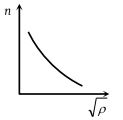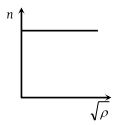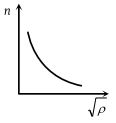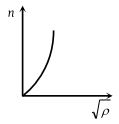NEET Questions Solved

The correct graph between the frequency n and square root of density (ρ) of a wire, keeping its length, radius and tension constant, is

(1)(2)(3)(4)(3) We know frequency $n=\frac{p}{2l}\sqrt{\frac{T}{\pi {r}^{2}\rho }}$$n\propto \frac{1}{\sqrt{\rho }}$

i.e., graph between n and $\sqrt{\rho }$ will be hyperbola.

Difficulty Level:

• 6%
• 39%
• 50%
• 6%